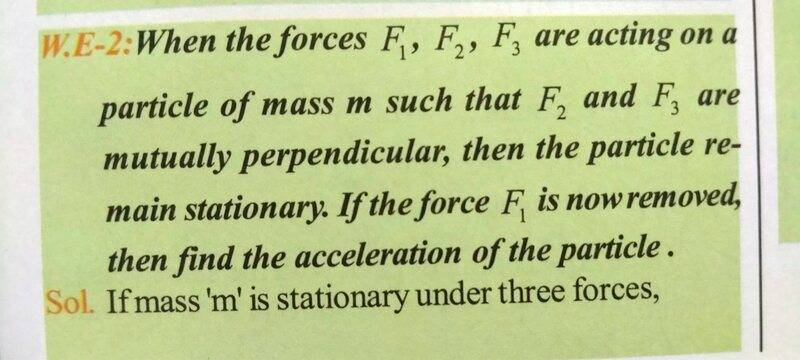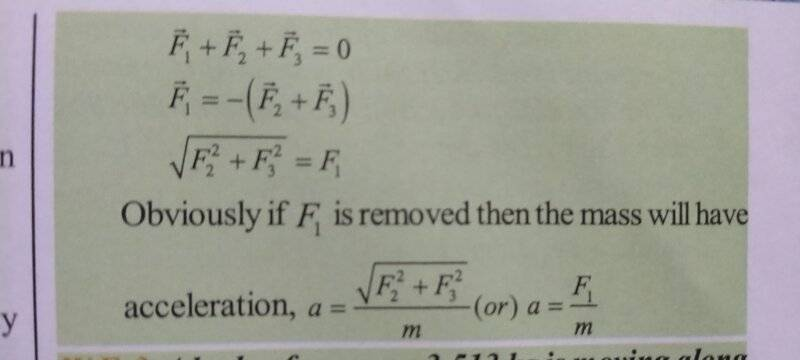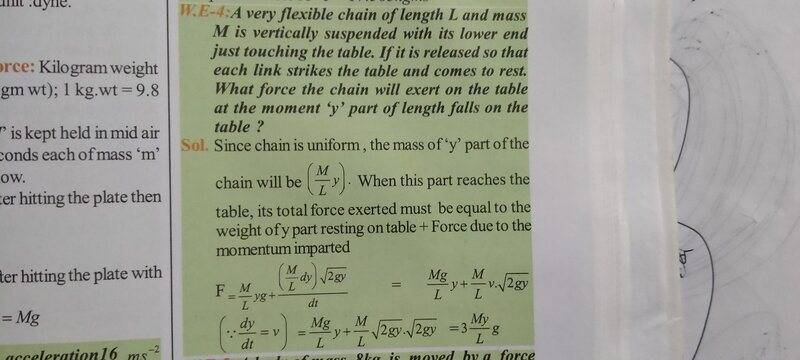# How is the acceleration proportional to the removed force?

• B
Gold MemberImage above is the question. Below image depicts solution.if F1 is removed then the acceleration of that mass must be sum of accelerations of remaining forces. Right??
But answer says that acceleration of that mass is equal to acceleration of F1. I don't understand it. Can someone explain it??

Last edited by a moderator:

But answer says that acceleration of that mass is equal to acceleration of F1. I don't understand it. Can someone explain it??
If initial acceleration is 0 and you remove a force F, then the acceleration is -F/m (opposite to the removed force).

•Mr.Husky
Homework Helper
The sum of the remaining forces must be -F1, otherwise the mass would have been accelerating before F1 was removed.
If you include the direction in the acceleration, it is A=-F/m.

•Mr.Husky
Gold Member
The sum of the remaining forces must be -F1, otherwise the mass would have been accelerating before F1 was removed.
I don't like their answer. In should be a=-F1/m.
Oh I got it. It is just a typo. Right??

Gold Member
If initial acceleration is 0 and you remove a force F, then the acceleration is -F/m (opposite to the removed force).

Homework Helper
Oh I got it. It is just a typo. Right??
No. They are not including the direction vector. Note the F1 with and without the direction arrow on top.

•Mr.Husky
Gold Member
No. They are not including the direction vector. Note the F1 with and without the direction arrow on top.
I forget about the vector notation. Sorry for it.
By the way I also have another example can you explain it??
What I don't understand in the following example is how did they got to know that the total force exerted must be equal to the weight of y part resting on table+ force due to the momentum impartedGold Member
By the way the text I am using is prepared by my school and is it worth going through these types of questions (there are about 300 of them per chapter) ??
Can you compare the difficulty of questions of this book to kleppner kolenkow classical mechanics book?

Homework Helper
What I don't understand in the following example is how did they got to know that the total force exerted must be equal to the weight of y part resting on table+ force due to the momentum imparted
If I throw a link against the wall, it will exert a momentary force on the wall.
If I throw a link onto a table, it will exert a momentary force and then come to rest on the table.
A link resting on the table exerts a downward force on the table.

Regarding the Physics books: It's been decades since I took a Physics course.

•Mr.Husky
Gold Member
If I throw a link against the wall, it will exert a momentary force on the wall.
If I throw a link onto a table, it will exert a momentary force and then come to rest on the table.
A link resting on the table exerts a downward force on the table.

Regarding the Physics books: It's been decades since I took a Physics course.
Now I am slowly understanding the answer.
Can you rate difficulty of the questions generally??

Homework Helper
It would be on par for introductory Physics - either High School or College PH101.

Gold Member
Can anybody compare the difficulty of the question to that of kleppner and kolenkow. Because I am not sure whether to go on with this book or should I change to kk which explains theory brilliantly.

Gold Member
If I throw a link against the wall, it will exert a momentary force on the wall.
If I throw a link onto a table, it will exert a momentary force and then come to rest on the table.
A link resting on the table exerts a downward force on the table.

Regarding the Physics books: It's been decades since I took a Physics course.
Scott can you explain how they got the momentum in the first equation. And also why did they considered momentum rather than downward force??

Gold Member
Scott can you explain how they got the momentum in the first equation. And also why did they considered momentum rather than downward force??
Thanks Scott for helping me!